# Marriage sttus

In our city, there are 3/5 of the women married to 2/3 of the men. Find what part of the population is free.

p =  0.3684

### Step-by-step explanation:Did you find an error or inaccuracy? Feel free to write us. Thank you!Tips to related online calculators
Need help to calculate sum, simplify or multiply fractions? Try our fraction calculator.
Do you have a linear equation or system of equations and looking for its solution? Or do you have a quadratic equation?

## Related math problems and questions:

• Freedom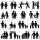In the city are 3/9 of women married for 3/6 men. What proportion of the townspeople is free (not married)? Express as a decimal number.
• The city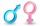In the city is 2/4 of women married to 3/4 men. What percentage of townspeople is single (not married)?
• The buildingThere are 45 people living in building, out of these 3/5 are men,2/5 are women . find the number of men and women in the building.
• Two fifth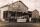Two fifth of the worker in a factory are men. The rest are women. If there 28 men . how many more women than men are there?
• Find unknown 2Find unknown denominator: 2/3 -5/? = 1/4
• What is 9What is the value of x in the proportion 2 and one-fourth over x = 1 and one-half over 3 and three-fifths? 2 and two-fifths 5 and two-fifths 8 and 1 over 10 12 and 3 over 20
• Two thirdsTwo thirds of the unknown number is 8/7. What is 7/3 of this number?
• If-then equationIf 1/2 + 2/5s = s - 3/4 what is the value of s?
• TogetherIf 8 men, 10 women, 16 children collects ₹1024 in 4 days, how many days will be required for 6 men, 5 women and 4 boys to collect ₹768? (₹ is Indian Rupee)
• Two numbers 11The sum of two rational numbers is (-2). If one of them is 3/5, find the other.
• Even / odd numbers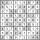a / Using variable n write two consecutive odd numbers. b / the sum of three consecutive odd numbers is 333. What are this numbers?
• Fractions and mixed numerals(a) Convert the following mixed numbers to improper fractions. i. 3 5/8 ii. 7 7/6 (b) Convert the following improper fraction to mixed number. i. 13/4 ii. 78/5 (c) Simplify these fractions to their lowest terms. i. 36/42 ii. 27/45 2. evaluate following ex
• Value of expressionX=2, y=-5 and z=3 what is the value of x-2y?
• Interior anglesCalculate the interior angles of a triangle that are in the ratio 2: 3: 4.
• Orchard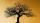3/5 of the trees in the orchard are apples, 1/3 are cherries and the remaining five trees are pear. How many trees are in the orchard?
• Trip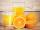On the trip drank 3/10 of pupils tea, 2/5 cola, 1/4 mineral water and remaining 3 juice. How many students were on the trip?
• Ratio of volumesIf the heights of two cylindrical drums are in the ratio 7:8 and their base radii are in the ratio 4:3. What is the ratio of their volumes?